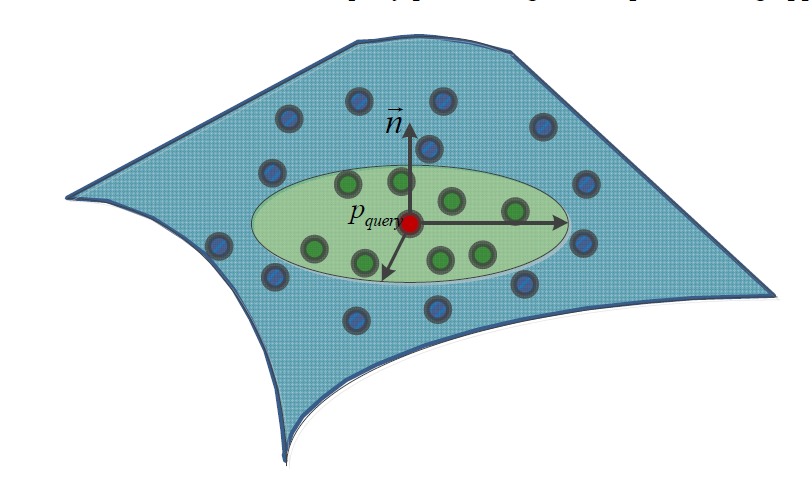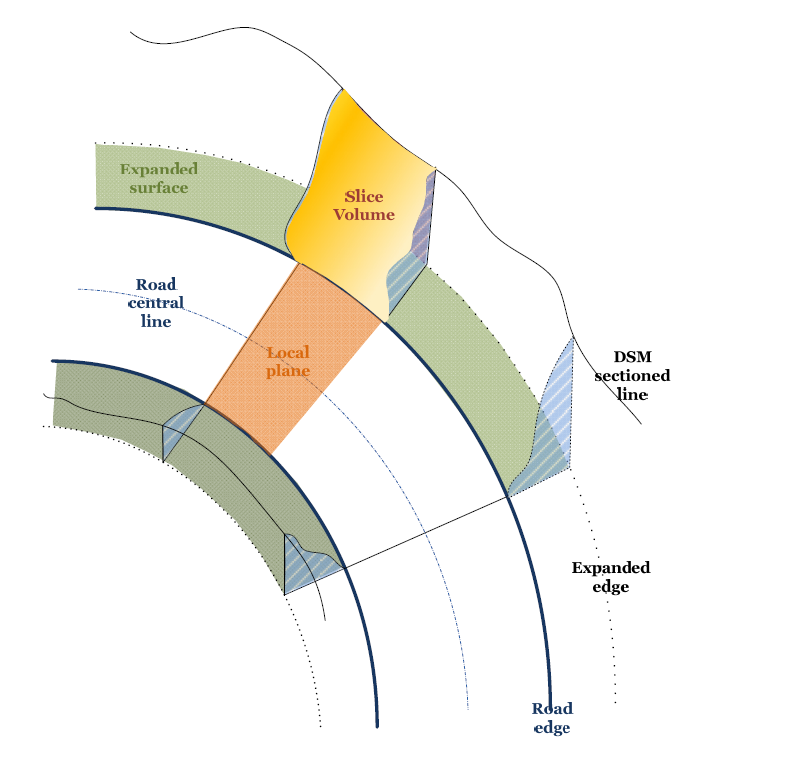## TU ANUNCIO / YOUR PUBLICITY

AQUÍ PODRÍA ESTAR TU ANUNCIO: / HERE COULD BE YOUR AD E-mail

## Article sponsored by Extraco, Misturas, Lógica, Enmacosa and Ingeniería Insitu inside the SITEGI project, cofinanced by the CDTI. (2012)

Autores / Authors : Jinhu Wang 1,*, Higinio González-Jorge 2, Roderik Lindenbergh 1, Pedro Arias-Sánchez 2 and Massimo Menenti

1. Department of Geoscience and Remote Sensing, Delft University of Technology, Building 23, Stevinweg 1, Post Box 5048, 2628 CN Delft, The Netherlands; E-Mails: r.c.lindenbergh@tudelft.nl (R.L.); m.menenti@tudelft.nl (M.M.)
2. 2 Department of Natural Resources and Environmental Engineering, School of Mining Engineering, University of Vigo, E-36310 Vigo, Spain; E-Mails: higiniog@uvigo.es (H.G.-J.); parias@uvigo.es (P.A.-S.)
* Author to whom correspondence should be addressed; E-Mail: jinhu.wang@tudelft.nl;

### Abstract:

Roads play an indispensable role as part of the infrastructure of society. In recent years, society has witnessed the rapid development of laser mobile mapping systems (LMMS) which, at high measurement rates, acquire dense and accurate point cloud data.

This paper presents a way to automatically estimate the required excavation volume when widening a road from point cloud data acquired by an LMMS. Firstly, the input point cloud is down-sampled to a uniform grid and outliers are removed. For each of the resulting grid points, both on and off the road, the local surface normal and 2D slope are estimated.

Normals and slopes are consecutively used to separate road from off-road points which enables the estimation of the road centerline and road boundaries. In the final step, the left and right side of the road points are sliced in 1-m slices up to a distance of 4 m, perpendicular to the roadside. Determining and summing each sliced volume enables the estimation of the required excavation for a widening of the road on the left or on the right side. The procedure, including a quality analysis, is demonstrated on a stretch of a mountain road that is approximately 132 m long as sampled by a Lynx LMMS. The results in this particular case show that the required excavation volume on the left side is 8% more than that on the right side. In addition, the error in the results is assessed in two ways. First, by adding up estimated local errors, and second, by comparing results from two different

datasets sampling the same piece of road both acquired by the Lynx LMMS. Results of both approaches indicate that the error in the estimated volume is below 4%. The proposed method is relatively easy to implement and runs smoothly on a desktop PC. The whole workflow of the LMMS data acquisition and subsequent volume computation can be completed in one or two days and provides road engineers with much more detail than traditional single-point surveying methods such as Total Station or GPS profiling. A drawback is that an LMMS system can only sample what is within the view of the system from the road.

### 1. Introduction

#### 2. Methodology

This section explains the procedure for estimating the amount of roadside material that has to be removed for road widening. It consists of five steps. (1) Point cloud data preprocessing; the original point cloud data have a very high point density and need to be downsampled before processing. Additionally, outliers are removed, and a Digital Surface Model (DSM) is generated; (2) Surface normal estimation; (3) Slope and aspect estimation; (4) Road detection and segmentation; taking the normal and slopes as estimated in steps (2) and (3) as input, an automatic iterative filtering approach is used to segment the road points from the downsampled point cloud data; (5) Volume computation; the material volume that needs to be moved to widen the road is computed based on step (4). A flowchart of the procedure is shown in Figure 1.

#### 2.1. Point Cloud Data Preprocessing

The raw point cloud data have a very high point density, so for quick and efficient processing, downsampling is a necessity. The approach followed here is to represent the point cloud data by voxels [21–23]. In this paper, a uniform-sized voxel filtering approach was used, as implemented in the Point Cloud Library (PCL) . The main concept of the approach is to create a 3D voxel using a given voxel size. All points in a voxel are represented by their centroid . The outliers of the downsampled point cloud data have to be removed before estimating geometric features from the point cloud data. In this procedure, the query point neighbourhood concept is introduced, as shown in Figure 2. Here, P represents the set of points within radius Rquery of query point pquery, so P is defined as:

where p(i) represents the individual points from the point cloud.Figure 1. Overall methodology of data processing.Figure 2. Neighbourhood of a query point within a certain radius.
Outliers are the measurements located at jump edges or discontinuous boundaries where there should be no points . There is abundant literature on the approaches used to remove outliers [25–27]. The approach used in this paper is based on a statistical analysis of each point’s neighbourhood [28,29]. For each point pquery Îcalculated. After that, for each point in the point cloud, the mean distance and standard deviation of the distances to their k nearest neighbours are determined. The main objective is to retain only those points whose mean distance to the closest k neighbors is similar to the average mean distance. As this describes a measure of the underlying point cloud density surrounding a point, the criterion for keeping a point is simply formulated as follows:

where, α is a desired density restrictiveness factor, while 􀟤􀯞 and 􀟪􀯞 are the mean and standard deviation of the distance from a query point to its neighbors respectively, and 􀜲􀗛 is the set of remaining points.

#### 2.2. Local Surface Normal Estimation

The surface normal at a discrete point is a vector perpendicular to the tangential plane of the local surface at that point. Various methods exist to estimate a normal at a certain point in 3D point cloud data [29–33]. The simplest is based on 3D plane fitting. With this method, the problem of determining the normal of a point becomes a least-square 3D plane estimation problem for a suitable spatial neighbourhood. Figure 3 depicts the concept of normal estimation in discrete 3D point cloud data.

When estimating a local normal using least-squares, the goal is to approximate the tangent plane at a point of interest and take that plane’s normal. The best fitting plane is obtained by determining those planar parameters that minimize the squared distances between suitable neighbouring points and the plane to be estimated. Suppose we have a point of interest with Euclidean coordinates (x, y, z)set of k neighbouring points. The least-squares method yields a normal vector (nx, ny, nz)which the error is minimized.Figure 3. Normal estimation at a query point using a local plane fitting approach

#### 2.3. Local Slope Computation

The 2D slope, also known as the 2D gradient, is the vector field of a surface. The vector direction points in the direction of the greatest change in height, and the vector’s magnitude is equal to the rate of change. Based on the previously generated gridded DSM, the height at every grid point is interpolated from neighbouring points by inverse distance interpolation with power 2. If the grid size is d(grid), then the slope Si in the direction of each of the eight neighboring grid cells is given by:

Here, H(i) is the elevation of the i-th neighbour of the query point, and hquery is the elevation of the query point itself. The variable di is the distance between the i-th neighbour and the query point. Note that di is the square root of the grid size in diagonal direction.

Based on the normal vectors and slopes that were computed at each point in the previous steps, an
automatic iterative point cloud data filtering approach is used to detect road surface points from the
point cloud data. The main steps are as follows:
• Input an initial grid and window size;
• Generate a virtual reference 3D gridded layer. The elevation of each grid point is interpolated from its neighbouring points, and also the grid point’s normal as unit vector and its direction to zenith. Based on this layer, a window of predefined size is created to move over the grid and point cloud;
• In the current moving window, compare for each grid point the 3D layer grid elevation and the normal vector direction with that of the point cloud; Calculate the height and angle differences between the 3D layer and the point cloud to verify if the differences are beyond the threshold;
• If the difference is less than the distance and direction threshold, then the point is accepted as a road point, else the point is regarded as off-road point;
• Go to step (2) and generate a new virtual layer using a smaller grid size, then iteratively process the point cloud again;
• The loop ends when the grid size reaches a pre-defined smallest size.

#### 2.5. Calculation of Excavation Volume

In this step, based on the segmented road points from step 4, the road’s outlines are determined.
First, a local feature descriptor is defined based on the neighbourhood concept.Figure 4. Illustration of local neighbourhood feature descriptor.
After the road outline is determined, the road central line is estimated based on the location of the
road edges. Now suppose the road needs to be widened to four lanes. As a consequence, a certain
As shown in Figure 5, roadsides were divided into slices. For each slice, the volume is computed.
Summing all of the sliced volumes together gives the total volume that needs to be excavated or filled.
Note that the sign of a sliced volume indicates whether material needs to be removed (positive sign) or
added (negative sign). Based on the road central line and edge lines, as well as the expanded width of
the road, the expanded edge points are found.Figure 5. Computation of excavation volume.Figure 6. Slice volume computational geometry.